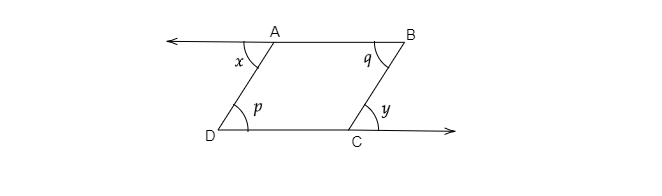"
">

# In the given figure, check if $x+y=p+q$."

Given: In the given figure two transversals intersect two parallel lines $AB$ and $CD$.

To do: To check if $x+y=p+q$.

Solution:As known, when a transversal intersects two parallel lines, alternative interior angles are equal.

$\Rightarrow \angle x=\angle p\ ......\ ( i)$

And similarly, $\angle y=\angle q\ .....\ ( ii)$

On adding $( i)$ and $( ii)$,

$\angle x+ \angle y=\angle x+\angle y$

Updated on: 10-Oct-2022

67 Views